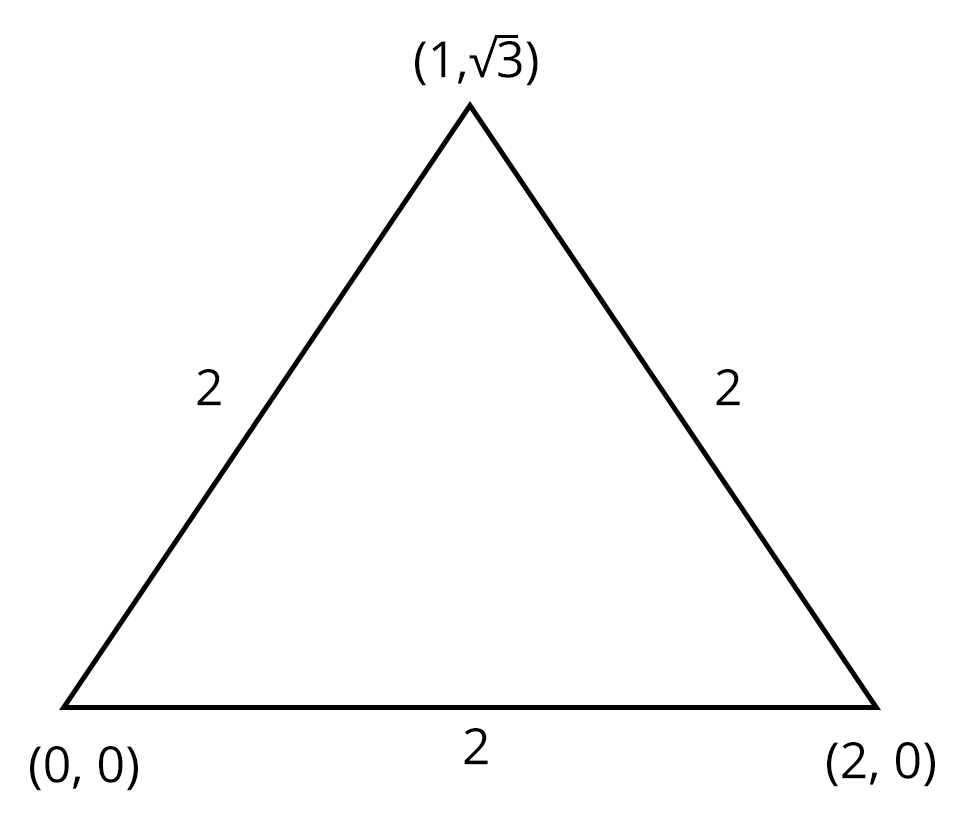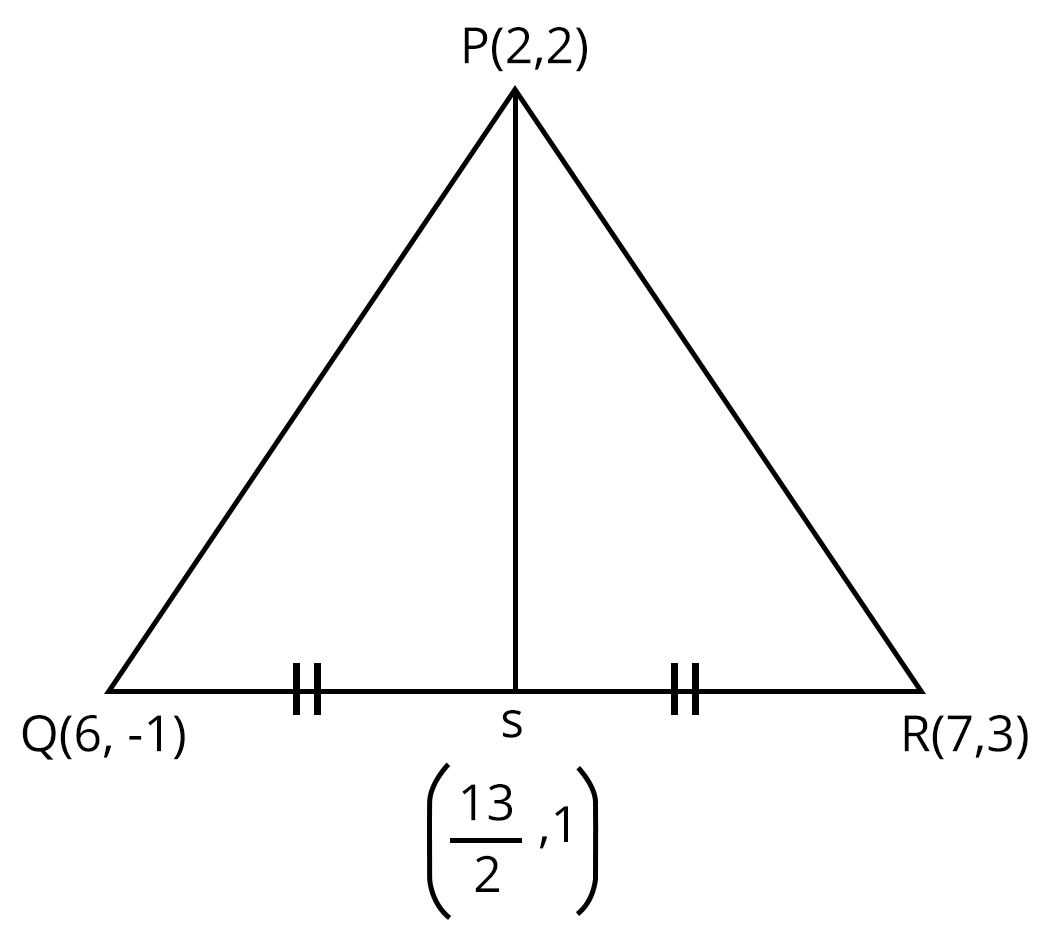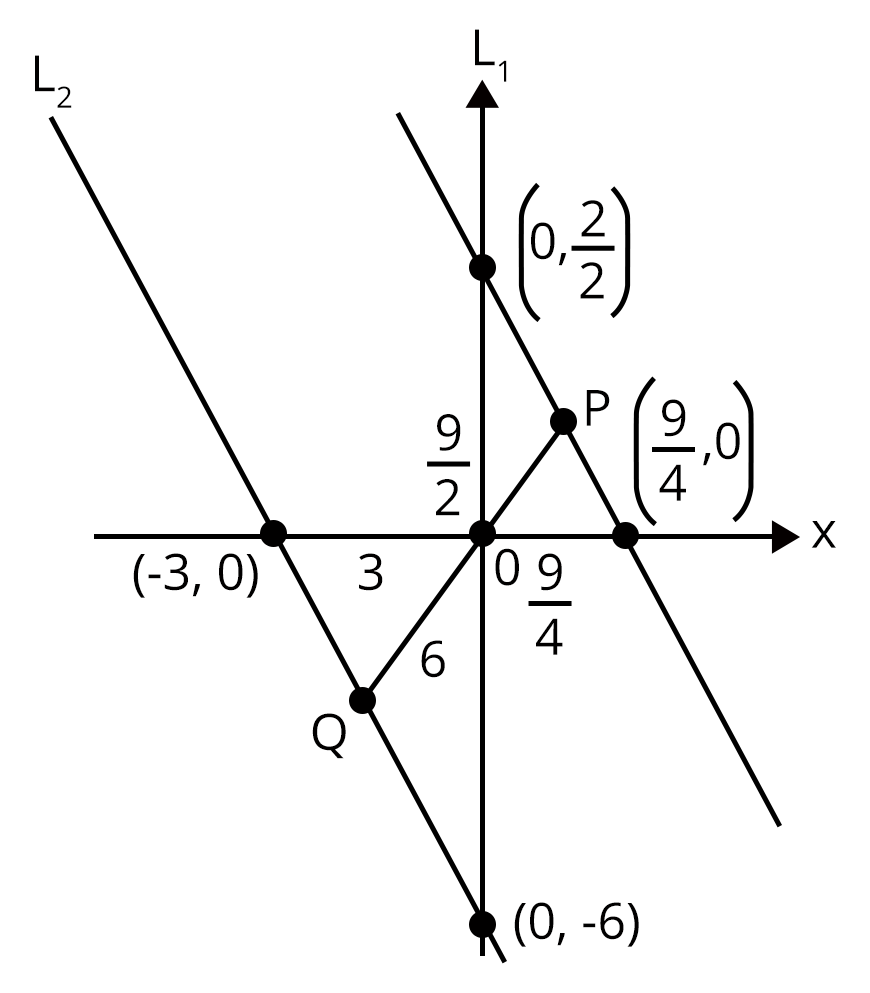# JEE - Analytic Geometry

Get plus subscription and access unlimited live and recorded courses## Introduction to Analytic Geometry

Analytic Geometry is a branch of algebra that deals with the modelling of geometrical objects such as lines, points, curves, and so on. It is a major invention of Descartes and Fermat. It is a mathematical subject in which problems are solved using algebraic symbolism and methods. It establishes the mathematical equations' relationship with geometric curves. "Coordinate Geometry" is a word that is sometimes used to refer to analytic geometry.

### Important Terms in Algebra

• Planes

• Cartesian Plane

• Distance formula

• Section Formula

• Direction ratios

• Locus

### What is Analytical Geometry

Analytic geometry is a branch of Mathematics in which the position of a point on a plane can be determined using an ordered pair of numbers known as coordinates. This is also known as Cartesian geometry or coordinate geometry. Analytic geometry differs from synthetic geometry in that it does not use coordinates or formulae. To solve difficulties, it is regarded as an axiom or assumption. However, in analytic geometry, the geometrical objects are defined using local coordinates. This geometry is also defined using algebra.

Both two-dimensional and three-dimensional geometry use coordinate geometry. It's a symbol for geometrical shapes. Let's look at some of the terms used in analytic geometry, such as:

• Plane

• Coordinates

### Coordinates

The two ordered pairs that specify the location of each given point in a plane are known as coordinates.

There are different types of coordinates in analytical geometry. Some of them are as follows:

• Cartesian Coordinates

• Polar Coordinates

• Cylindrical Coordinates

• Spherical Coordinates

### Cartesian Coordinates

The Cartesian coordinate system is the most well-known coordinate system, in which each point has an x-coordinate and a y-coordinate that expresses its horizontal and vertical positions, respectively. They're frequently addressed as a pair and referred to as (x, y). This method can also be used in three-dimensional geometry, where each point in Euclidean space is represented by an ordered triple of coordinates (x, y, z).

### Polar Coordinates

Each point in a plane is denoted by the distance 'r' from the origin and the angle θ  from the polar axis in polar coordinates.

### Cylindrical Coordinates

All points are represented in cylindrical coordinates by their height, radius from the z-axis, and angle projected on the xy-plane with regard to the horizontal axis. The letters h, r, and θ stand for height, radius, and angle, respectively.

### Spherical Coordinates

The distance from the origin (ρ), the angle projected on the xy-plane with regard to the horizontal axis (θ), and another angle with respect to the z-axis (φ) are all used to describe a point in space in spherical coordinates.

### Analytic Geometry Formulae in Two dimension

• Distance Formula: Let the two points be A and B, having coordinates to be $\left ( x_{1}, \,\ y_{1} \right )$ and $\left ( x_{2}, \,\ y_{2} \right )$, respectively. Thus, the distance between two points is given as:

$\Rightarrow d = \sqrt{\left ( y_{2}-y_{1} \right )^{2}+\left ( x_{2}- x_{1} \right )^{2}}$

• Section Formula: Let two lines A and B have coordinates $\left ( x_{1}, \,\ y_{1} \right )$, and $\left ( x_{2}, \,\ y_{2} \right )$, respectively. If the point P divides the two lines in the ratio of m:n, then the coordinates of P is given by,

When the ration m : n is internal,

$P\left ( x, \, y \right )= \left ( \frac{mx_{2}+nx_{1}}{m+n}, \, \frac{my_{2}+ny_{1}}{m+n} \right )$

When the ratio m : n is external,

$P\left ( x, \, y \right )= \left ( \frac{mx_{2}-nx_{1}}{m-n}, \, \frac{my_{2}-ny_{1}}{m-n} \right )$

• Midpoint Formula: Let A and B are some points in a plane, which is joined to form a line, having coordinates $\left ( x_{1}, \,\ y_{1} \right )$ and $\left ( x_{2}, \,\ y_{2} \right )$, respectively. Suppose P(x, y) is the midpoint of the line connecting the point A and B, then its formula is given by,

$P\left ( x, y \right )= \left ( \frac{x_{1}+x_{2}}{2} , \frac{y_{1}+y_{2}}{2} \right )$

• Angle Formula: Let two lines have slope $m_{1}$ and $m_{2}$ and θ is the angle formed between the two lines A and B, which is represented as:

$tan\theta = \left|\frac{m_{1}-m_{2}}{1+ m_{1}m_{2}} \right|$

### Analytic Geometry in Three Dimensions

We examine real-number triples (a, b, c) and refer to this set as a three-dimensional number space, denoted by R'. Coordinates refer to all of the elements in a triple.

Let's look at how a geometric space represents a three-dimensional number space.

Consider three mutually perpendicular lines intersecting at point O in three-dimensional space. Starting at 0, these lines are designated as coordinate axes, with identical number scales set up on each of them.

### Solved Examples

Example 1: The maximum value of $y = \sqrt{\left ( x-3 \right )^{2}+\left ( x^{2}-2 \right )^{2}}-\sqrt{x^{2}+\left ( x^{2}-1 \right )^{2}}$.

1. 3

2. $\sqrt{10}$

3. $2\sqrt{5}$

4. None of these

Solution:(b) let y = f(x) = $\sqrt{\left ( x-3 \right )^{2}+\left ( x^{2}-2 \right )^{2}}-\sqrt{x^{2}+\left ( x^{2}-1 \right )^{2}}$

Note that the first radical sign describes the distance between $P(x, x^{2})$ and A(3, 2) whereas the second radical sign describes the distance between  $P(x, x^{2})$ and B(0, 1).

Now $PA - PB \leq AB$ for possible positions of P.

Hence, $f(x)_{max} =$ distance between AB $= \sqrt{9+1}=\sqrt{10}$

Hence, option b is the correct answer.

Example 2: Number of values of $\alpha$ such that the points $\left ( \alpha , 6 \right )$, ( -5, 0), and (5, 0) form an isosceles triangle is

1. 4

2. 5

3. 6

4. 7

Solution: (b)

Here, we have $A\left ( \alpha ,6 \right )$, B(-5, 0), and C(5, 0).

If AB = AC, we get $\alpha = 0$.

Hence, we get one value of c,

If AC = BC, we get $\alpha = -3, 13$

$\alpha$ can take 5 values 0, 3, -3, 13, -13.

Hence, option (b) is the correct answer.

### Solved Problems of Previous Year Question

1. The incentre of the triangle with vertices $(1, \sqrt{3})$, (0, 0), and (2, 0) is

1. $\left ( 1, \frac{\sqrt{3}}{2} \right )$

2. $\left ( \frac{2}{3}, \frac{1}{\sqrt{3}} \right )$

3. $\left ( \frac{2}{3}, \frac{\sqrt{3}}{2} \right )$

4. $\left ( 1, \frac{1}{\sqrt{3}} \right )$

Ans: (d) here, slides are equal.

So, it is an equilateral triangle.

Thus, the incentre is centroid.Incentre = $\left ( \frac{0+2+1}{3}, \frac{0+0+\sqrt{3}}{3} \right )$

$\Rightarrow \left ( 1, \frac{1}{\sqrt{3}} \right )$

Hence, option (d) is the correct answer.

2. Let PS be the median of the triangle with vertices P(2, 2), Q(6,-1), and R(7,3). The equation of the line passing through (1,-1) and parallel to PS is

1. 2x - 9y -7 = 0

2. 2x - 9y -11 = 0

3. 2x + 9y -11 = 0

4. 2x + 9y + 7 = 0Here, midpoint of QR = (13/2, 1)

Now, slope of PS, $m_{ps}= \frac{2-1}{2-\frac{13}{2}}= \frac{1}{-\frac{9}{2}}= -\frac{2}{9}$

Passes through (1, -1)

$\Rightarrow y +1 = -\frac{2}{9}\left ( x - 1 \right )$

$\Rightarrow 2x + 9y + 7= 0$

3. A straight line through the origin O meets the parallel lines 4x + 2y = 9 and 2x + y + 6 = 0 at points P and Q, respectively. Then the point O divides the segment PQ in the ratio

1. 1 : 2

2. 3 : 4

3. 2 : 1

4. 4 : 3

Ans:

$L_{1} : 4x + 2y = 9$

$L_{2} : 2x + y + 6 = 0$Perpendicular distance anyline passing through origin

$\Rightarrow \frac{9}{4}: 3 \Rightarrow 3 : 4$

$\Rightarrow \frac{9}{2}: 6 \Rightarrow 3 : 4$

$\Rightarrow \frac{9}{2\sqrt{5}} : \frac{6}{\sqrt{5}}\Rightarrow 3 : 4$

### Practice Question

1. The number of triangles which are obtuse and which have the points (8, 9), (8, 16), and (20, 25) as the feet of perpendiculars drawn from the vertices on the opposite sides is

1. 0

2. 1

3. 2

4. 3

2. If A(5, 2), B(10, 12), and P(x, y) is such that AP/PB = 3/2, then the internal bisector of $\angle APB$ always passes through

1. (20, 32)

2. (8, 8)

3. (8,-8)

4. (-8, -8)

### Conclusion

In this chapter, we have elaborated on concepts and solutions to questions on the topic of Analytic Geometry.  Everything you're looking for is available in a single location. Students can carefully read through the concepts, definitions, and questions in the PDFs, which are also free to download, and understand the concepts used to solve these questions. This will be extremely beneficial to the students in their exams.

See More## JEE Advanced Important Dates

View All Dates
JEE Advanced 2022 exam date and revised schedule have been announced by the NTA. JEE Advanced 2022 will now be conducted on 28-August-2022, and the exam registration closes on 11-August-2022. You can check the complete schedule on our site. Furthermore, you can check JEE Advanced 2022 dates for application, admit card, exam, answer key, result, counselling, etc along with other relevant information.
See More
View All Dates## JEE Advanced Information

Application Form
Eligibility Criteria
Reservation Policy
IIT Bombay has announced the JEE Advanced 2022 application form release date on the official website https://jeeadv.ac.in/. JEE Advanced 2022 Application Form is available on the official website for online registration. Besides JEE Advanced 2022 application form release date, learn about the application process, steps to fill the form, how to submit, exam date sheet etc online. Check our website for more details.## JEE Advanced Syllabus

View JEE Advanced Syllabus in Detail
It is crucial for the the engineering aspirants to know and download the JEE Advanced 2022 syllabus PDF for Maths, Physics and Chemistry. Check JEE Advanced 2022 syllabus here along with the best books and strategies to prepare for the entrance exam. Download the JEE Advanced 2022 syllabus consolidated as per the latest NTA guidelines from Vedantu for free.
See More
View JEE Advanced Syllabus in Detail## JEE Advanced 2022 Study Material

View all study material for JEE Advanced
JEE Advanced 2022 Study Materials: Strengthen your fundamentals with exhaustive JEE Advanced Study Materials. It covers the entire JEE Advanced syllabus, DPP, PYP with ample objective and subjective solved problems. Free download of JEE Advanced study material for Physics, Chemistry and Maths are available on our website so that students can gear up their preparation for JEE Advanced exam 2022 with Vedantu right on time.
See More
All
Chemistry
Maths
Physics
See All## JEE Advanced Question Papers

see all
Download JEE Advanced Question Papers & ​Answer Keys of 2022, 2021, 2020, 2019, 2018 and 2017 PDFs. JEE Advanced Question Paper are provided language-wise along with their answer keys. We also offer JEE Advanced Sample Question Papers with Answer Keys for Physics, Chemistry and Maths solved by our expert teachers on Vedantu. Downloading the JEE Advanced Sample Question Papers with solutions will help the engineering aspirants to score high marks in the JEE Advanced examinations.
See MoreView all JEE Advanced Important Books
In order to prepare for JEE Advanced 2022, candidates should know the list of important books i.e. RD Sharma Solutions, NCERT Solutions, HC Verma books and RS Aggarwal Solutions. They will find the high quality readymade solutions of these books on Vedantu. These books will help them in order to prepare well for the JEE Advanced 2022 exam so that they can grab the top rank in the all India entrance exam.
See More
Maths
NCERT Book for Class 12 Maths
Physics
NCERT Book for Class 12 Physics
Chemistry
NCERT Book for Class 12 Chemistry
Physics
H. C. Verma Solutions
Maths
R. D. Sharma Solutions
Maths
R.S. Aggarwal Solutions
See All## JEE Advanced Mock Tests

View all mock tests
JEE Advanced 2022 free online mock test series for exam preparation are available on the Vedantu website for free download. Practising these mock test papers of Physics, Chemistry and Maths prepared by expert teachers at Vedantu will help you to boost your confidence to face the JEE Advanced 2022 examination without any worries. The JEE Advanced test series for Physics, Chemistry and Maths that is based on the latest syllabus of JEE Advanced and also the Previous Year Question Papers.
See More## JEE Advanced 2022 Cut-Off

JEE Advanced Cut Off
IIT Bombay is responsible for the release of the JEE Advanced 2022 cut off score. The qualifying percentile score might remain the same for different categories. According to the latest trends, the expected cut off mark for JEE Advanced 2022 is 50% for general category candidates, 45% for physically challenged candidates, and 40% for candidates from reserved categories. For the general category, JEE Advanced qualifying marks for 2021 ranged from 17.50%, while for OBC/SC/ST categories, they ranged from 15.75% for OBC, 8.75% for SC and 8.75% for ST category.
See More## JEE Advanced 2022 Results

JEE Advanced 2022 Result - IIT Bombay has announced JEE Advanced result on their website. To download the Scorecard for JEE Advanced 2022, visit the official website of JEE Advanced i.e https://jeeadv.ac.in/.
See More
Rank List
Counselling
Cutoff
JEE Advanced 2022 State Wise Rank List - The respective state authorities started releasing the JEE Advanced 2022 state merit lists for admission to Engineering course. Check JEE Advanced 2022 state-wise rank list for the year 2022 here.## JEE Advanced Top Colleges

View all JEE Advanced 2022 Top Colleges
Want to know which Engineering colleges in India accept the JEE Advanced 2022 scores for admission to Engineering? Find the list of Engineering colleges accepting JEE Advanced scores in India, compiled by Vedantu. There are 1622 Colleges that are accepting JEE Advanced. Also find more details on Fees, Ranking, Admission, and Placement.
See More## FAQs on JEE - Analytic Geometry

FAQ

1. How do you find the intersecting point of the X-axis and Y-axis?

The initial point of intersection of the axis occurs at the origin where both the values of X and Y are represented as (x,y)= (0,0). Now if any two lines are drawn on the X/Y plane that are not parallel to each other, it is almost a certainty that the lines will intersect.

For instance, if you plot the x+2y=0 and x-2y=0 on a graph, these lines will intersect. But the question is at which point will they intersect each other. For finding the values of (x,y) at which both the lines intersect, you need to solve the equation of the lines, using a simultaneous linear equation.

2. What is the analytical geometry of three dimensions and its applications?

In three-dimensional space, we consider three mutually perpendicular lines intersecting in a point O and here we consider triples (a,b,c) which are real numbers and call this set a three-dimensional number space and denote it by R’.

Applications for analytic geometry are spread across a variety of fields and sciences. The application of analytical geometry in the engineering field and Physics is most mentionable among the others.  It is also essentially related to other mathematical fields like trigonometry and analytic geometry and calculus and analytic geometry Chapter 3.

Get a complete grasp on the formulas for analytic geometry and also the application of the analytical geometry field through in-depth analysis, explanations, sums, and comprehensive assistance and tutorials from the experts.## JEE Advanced Upcoming Dates28 Sep 20223 days remaining## Notice board

News
BlogTrending pages